# Equation + unit conversion - math problems

#### Number of problems found: 65

• Vertical rodThe vertical one meter long rod casts a shadow 150 cm long. Calculate the height of a column whose shadow is 36 m long at the same time.
• Train delay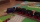Due to a breakdown, the train lost 16 minutes of standing on the track behind Brno. He "eliminated" this delay so that after the start, the 80 km long section went at a speed 10 km/h higher than originally planned. What speed was it and what was it suppos
• Beer tapping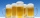When checking compliance with the beer tapping, it was found that 60% of the offered beers were underfilled. The others were fine. Thus, instead of 0.5 l, the volume was 4.4 dcl on average. What was the volume of one average underfilled beer?
• Two trainsThe train runs at speed v1 = 72 km/h. The passenger, sitting in the train, observed that a train long l = 75m in 3 s passed on the other track in the opposite direction. Calculate the speed of this train.
• Nutballs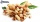The dough for nutballs contains, among other things, two basic raw materials: flour and nuts in a ratio of 2:1. How much flour and how many nuts are needed for 1 kg of dough if "other" is 100g?
• Angled cyclist turnThe cyclist passes through a curve with a radius of 20 m at 25 km/h. How much angle does it have to bend from the vertical inward to the turn?
• Cheetah vs antelope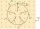When the cheetah began chasing the antelope, the distance between them was 120 meters. Although the antelope was running at 72km/h, the cheetah caught up with it in 12 seconds. What speed was the cheetah running?
• Accelerated motion - mechanicsThe delivery truck with a total weight of 3.6 t accelerates from 76km/h to 130km/h in the 0.286 km long way. How much was the force needed to achieve this acceleration?
• An investmentHow long will it take for an investment to double at a simple interest rate of 5.10% p. A. ? Express the answer in years and months, rounded up to the next month.
• Wood dividingWhich equation calculates the number of 1/3-foot pieces that can be cut from a piece of wood that is 7 feet long?
• CranberriesThey brought 65 kg of dried cranberries to the packing house. They made 150-gram and 200-gram packages from them. How many kilograms of cranberries did they use for the smaller 200 packages? How many 200 gram packages were there?
• GrandmotherMom walked out to visit her grandmother in a neighboring village 5km away and moved at a speed of 4km/h. An hour later, father drove down the same road at an average speed of 64km/h. 1) How long will take to catch mom die? 2) What is the approximate dista
• Granules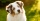Katy had two dogs. The first one ate a pack of granules a week and the other two times longer. Katka bought them two bags of granules. How long does it last for both dogs?
• Mushrooms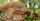Grandfather gathered fresh mushrooms. The fifth was wormwood, and it was thrown away, the other dried up. He obtained 720 grams of dried mushrooms. How many kilograms did the grandfather collect, and by drying the mushrooms they lost 75% of their weight?
• Two trainsThrough the bridge, long l = 240m, the train passes through the constant speed at time t1 = 21s. A train running along the traffic lights at the edge of the bridge passes the same speed at t2 = 9s. a) What speed v did the train go? b) How long did it take
• One kilogramThe apple weighs 125 grams and half apple. How many apples weigh 1 kilogram?
• Two shipsThe distance from A to B is 300km. At 7 am started from A to B a ferry with speed higher by 20 km/h than a ship that leaves at 8 o'clock from B to A. Both met at 10h 24min. Determine how far they will meet from A and when they reach the destination.
• The thirdThe one-third rod is blue, one-half of the rod is red, the rest of the rod is white and measures 8 cm. How long is the whole rod?
• Wiring 2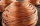Willie cut a piece of wire that was 3/8 of the total length of the wire. He cut another piece that was 9m long. The two pieces together were one half of the total length of the wire. How long was the wire before he cut it?
• Car driver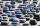The car driver is in town A and is scheduled to be in town B at a specified hour. If it travels at an average speed of 50km/h, it will arrive in city B 30 minutes later. But if he travels at an average speed of 70km/h, he would arrive half an hour earlier

Do you have an interesting mathematical word problem that you can't solve it? Submit a math problem, and we can try to solve it.

We will send a solution to your e-mail address. Solved examples are also published here. Please enter the e-mail correctly and check whether you don't have a full mailbox.

Please do not submit problems from current active competitions such as Mathematical Olympiad, correspondence seminars etc...

Do you have a linear equation or system of equations and looking for its solution? Or do you have quadratic equation?